• Slides: 14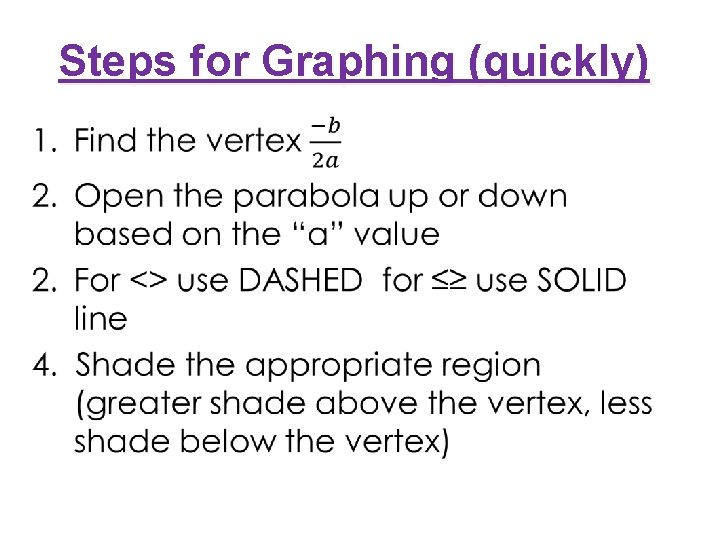Steps for Graphing (quickly) •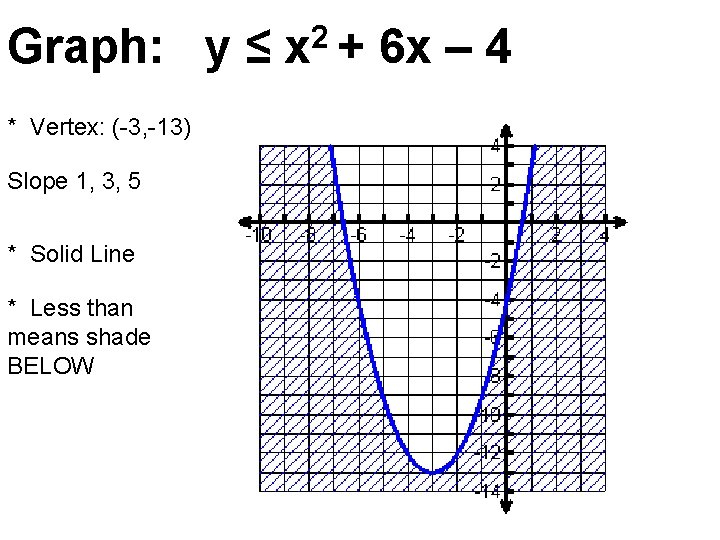Graph: y ≤ x 2 + 6 x – 4 * Vertex: (-3, -13) Slope 1, 3, 5 * Solid Line * Less than means shade BELOW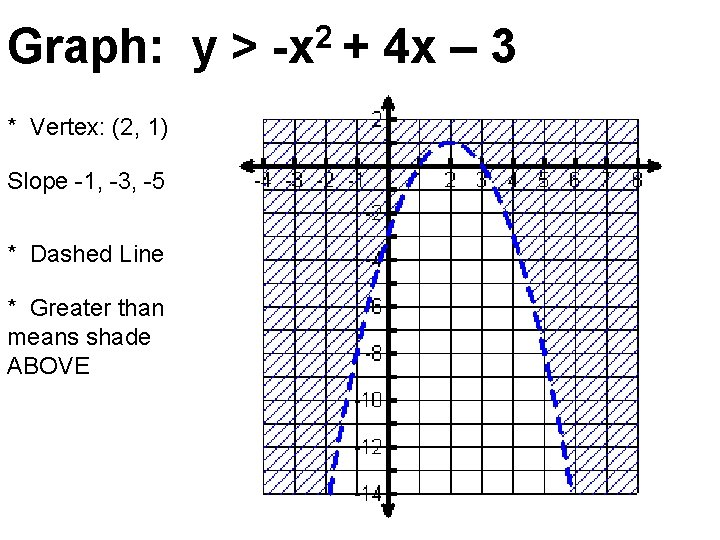Graph: y > -x 2 + 4 x – 3 * Vertex: (2, 1) Slope -1, -3, -5 * Dashed Line * Greater than means shade ABOVE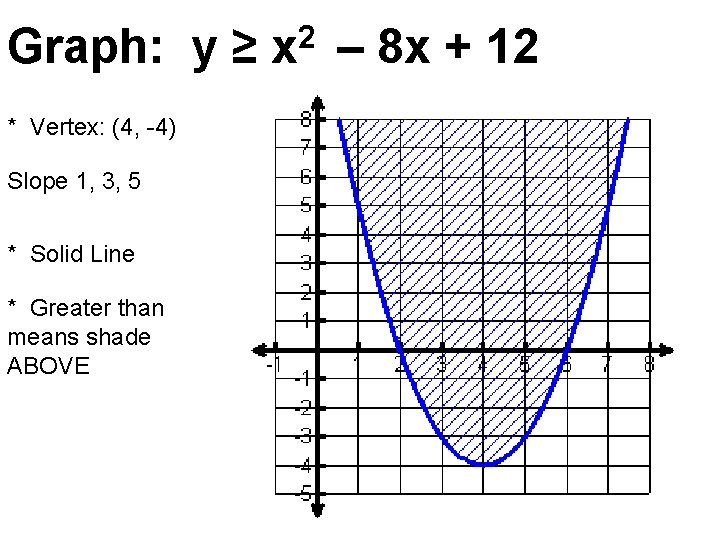Graph: y ≥ x 2 – 8 x + 12 * Vertex: (4, -4) Slope 1, 3, 5 * Solid Line * Greater than means shade ABOVE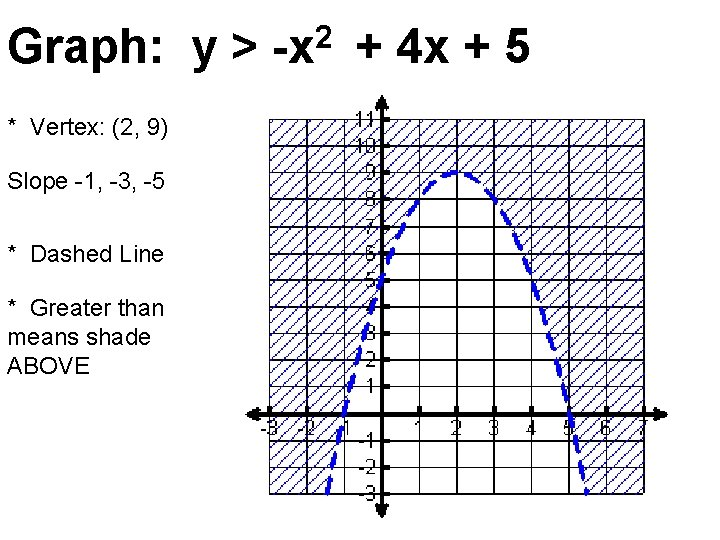Graph: y > -x 2 + 4 x + 5 * Vertex: (2, 9) Slope -1, -3, -5 * Dashed Line * Greater than means shade ABOVE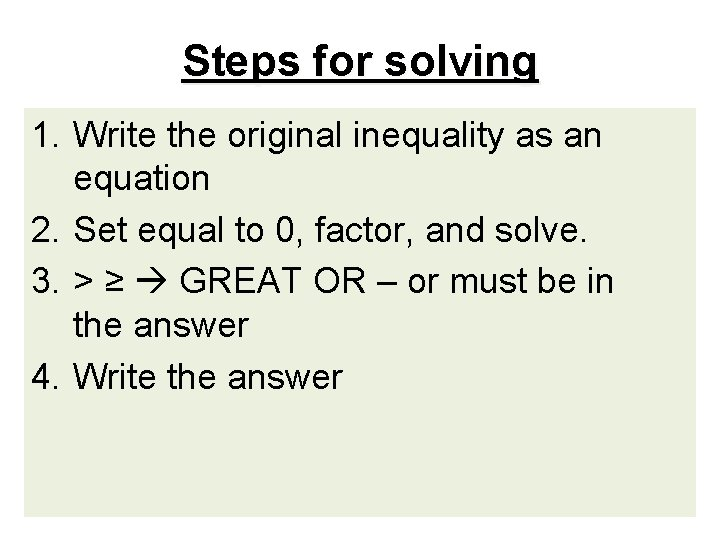Steps for solving 1. Write the original inequality as an equation 2. Set equal to 0, factor, and solve. 3. > ≥ GREAT OR – or must be in the answer 4. Write the answer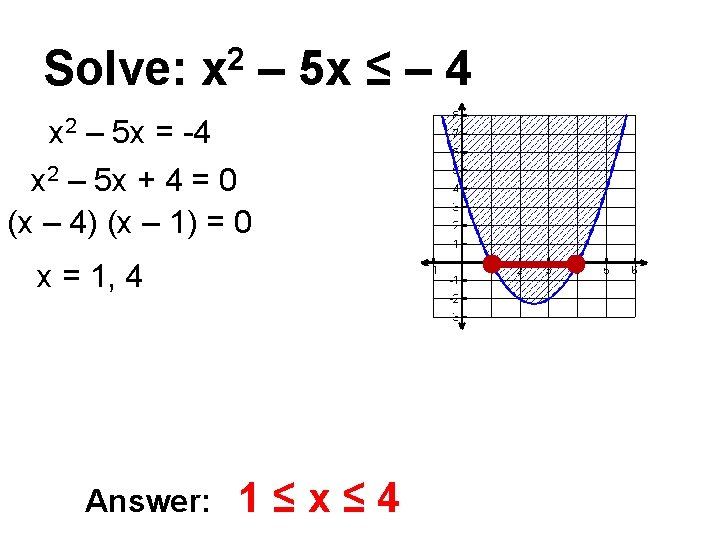Solve: 2 x – 5 x ≤ – 4 x 2 – 5 x = -4 x 2 – 5 x + 4 = 0 (x – 4) (x – 1) = 0 x = 1, 4 Answer: 1≤x≤ 4Solve: -x 2 + 7 x < 12 -x 2 + 7 x = 12 -x 2 + 7 x – 12 = 0 x 2 – 7 x + 12 = 0 (x – 4) (x – 3) = 0 x = 3, 4 Answer: x < 3 or x > 4What if it can’t factor? Graph it!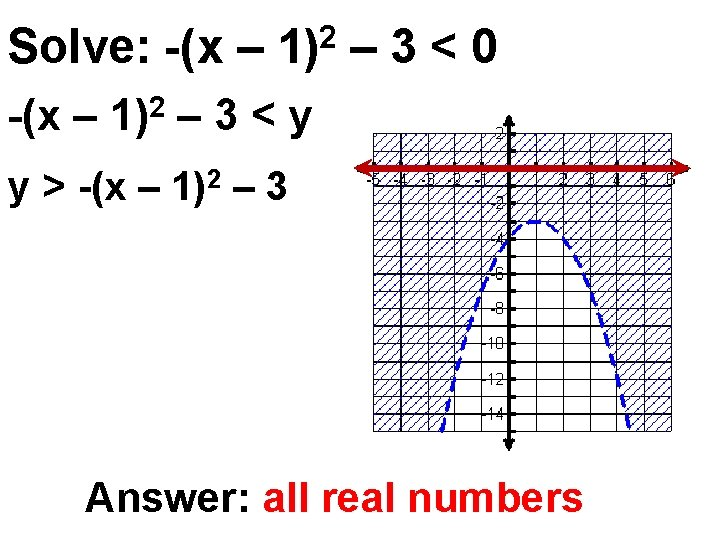Solve: -(x – 1)2 – 3 < 0 -(x – 1)2 – 3 < y y > -(x – 1)2 – 3 Answer: all real numbersSolve: x 2 + 4 ≤ 0 2 x +4≤y y≥ 2 x +4 Answer: no solution